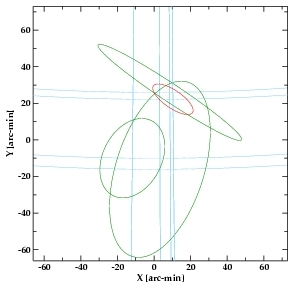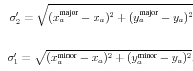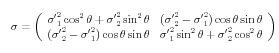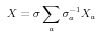## Source Position Errors in the Master Sources Table

### Summary

The position and 2-dimensional positional uncertainty of a source listed in the Master Sources Table represents the best estimate of the source position based on several independent measures, where the master source entry is the merged result of multiple observations of the same source. To determine the best estimate of the position of a source from previous independent estimates of its position, we employ a 2-dimensional optimal weighting formalism to statistically average the source positions resulting from the set of individual observations of the source. We decided to use this technique because it offers an improved estimate of source position where simple averaging fails, e.g. where the area defining the source position varies significantly from measure to measure. We express the uncertainties of the estimates in the form of error ellipses centered upon the estimated source positions.An example of input ellipses (green) and the combined ellipse (red).
The x and y values represent tangent plane coordinates.

The error ellipses that are combined to produce the "best estimate" error ellipse for the merged source entry result from the Chandra Multiwavelength Project (ChaMP) positional uncertainty relations, as described in the page "Source Position Errors in the Source Observations Table."

The use of the multivariate optimal weighting formalism in the Chandra Source Catalog, described below, represents its first application to astrophysical data, as it is based on an analysis of weapons targeting from a Master's thesis from the Naval Postgraduate School. For more information on this analysis and its use in the Chandra Source Catalog, see Joseph R. Orechovesky's Master's thesis "Single Source Error Ellipse Combination" and John Davis' CSC document "Combining Error Ellipses," respectively.

### Error Ellipses

#### Multivariate Optimal Weighting

The multivariate optimal weighting formalism used to combine error ellipses can be distilled to the following formula

where Xa represnts the ath estimate of the mean of the 2-dimensional source position, σa denotes the 2 x 2 covariance matrix associated with this estimate, and

#### Tangent Plane Projection

Before the covariance matrix σ may be computed, permitting the ellipses to be combined via the multivariate weighted sum, the error ellipses must be mapped from the celestial sphere onto a common tangent plane. The ath estimate of the source position is specified as a confidence-ellipse centered upon the celestial coordinate (αa, δa), with the major axis making an angle θ (-pi <= θ < pi) with respect to the local line of declination at the center of the ellipse. The tangent plane coordinates (xa, ya) of the center of the ath ellipse are

where pa is a unit-vector on the celestial sphere corresponding to the ath estimate of the celestial coordinate (αa, δa) defining the source position, given by

Similar equations give the end-point positions paminor and pamajor of the semi-minor and semi-major axes of each ellipse

where φaminor is the arc-length of the semi-minor axis and φamajor is that of the semi-major axis.

p0 denotes the position on the celestial sphere where a tangent plane is to be erected; it is taken to be the arithmetic mean of the ellipse centers pa , i.e.,

A coordinate system may be given to the tangent plane with the origin at p0 and orthonormal basis vectors ex and ey parallel to the local lines of right ascension and declination at p0, i.e.,

where (α0, δ0) are the celestial coordinates that correspond to p0.

The tangent plane coordinates that correspond to the end-point positions pamajor and paminor of the semi-major and semi-minor axes of the ellipse are denoted by (xamajor, yamajor) and (xaminor, yaminor). The lengths of the semi-major and semi-minor axes in the tangent plane are given by

respectively. Finally, the angle that the semi-major axis makes with respect to the local line of declination is

Armed with these relations, it is easy to compute the tangent plane projections of the error ellipses.

### Computing Covariance Matrices

Three of the parameters specifying the geometry of each projected error ellipse are the semi-major and semi-minor axis lengths, and the position angle θ that the major axis of the ellipse makes with respect to the tangent plane y axis. The semi-major and semi-minor axis lengths correspond to the 1σ confidence intervals along these axes. More specifically, in a basis whose origin is at the center of the ellipse, and whose y axis is along the major axis of the ellipse, the correlation matrix is

Here, σ'1 is the 1σ confidence value along the minor axis of the ellipse, and σ'2 is that along the major axis (σ'2 >= σ'1). The form of the covariance matrix σ in the unrotated system follows from

where R is a rotation matrix that transforms a vector X to X' = RX. Here, R is defined as

which yields

At this point, the lengths of the semi-major and semi-minor axes of the soure position error ellipses in the tangent plane, σ'1 and σ'2 defined at the end of the previous section, may be input to the covariance matrix σ above, permitting the error ellipses to be combined via the multivariate weighted sum.This process produces the geometric parameters of a combined 2-D error ellipse on the tangent plane (X), which are recorded in the "Position and Position Errors" fields err_ellipse_r0, err_ellipse_r1, and err_ellipse_ang in the Master Chandra Source Table.